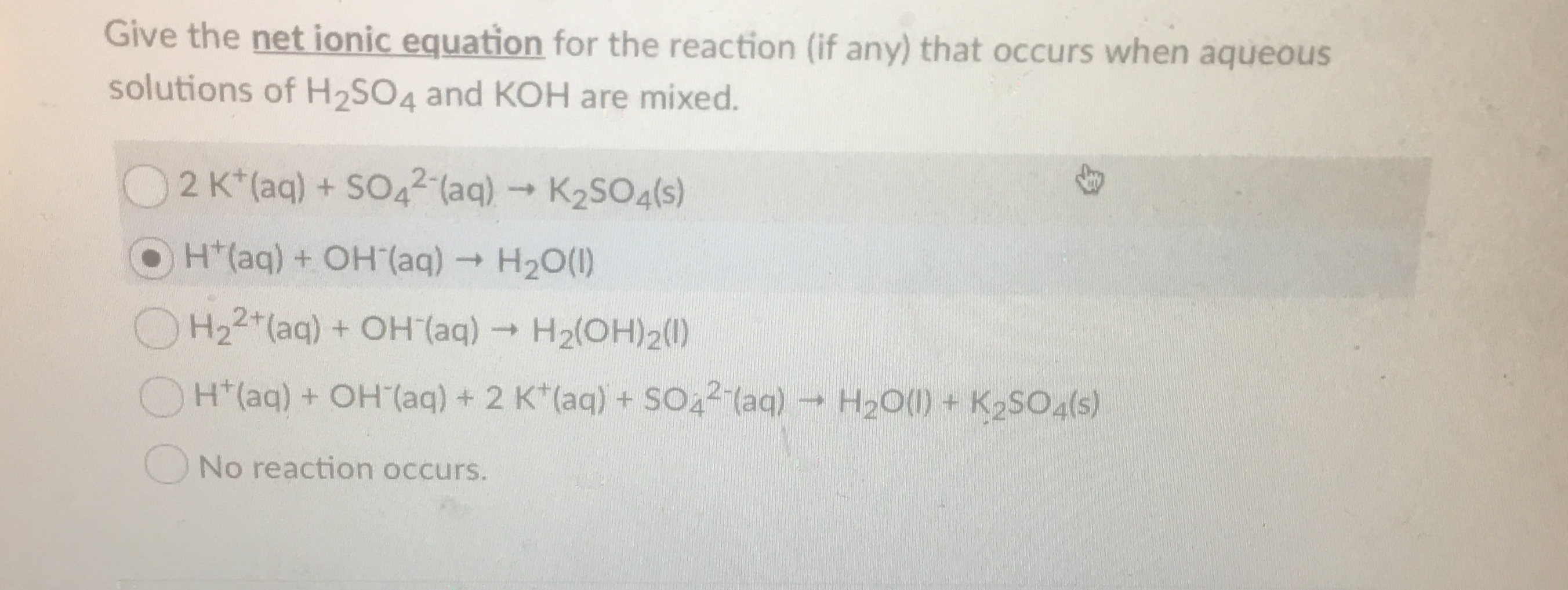# Give the net ionic equation for the reaction (if any) that occurs when aqueoussolutions of H2SO4 and KOH are mixed.2 K*(aq) + SO42(aq)K2SO4(s)OH(aq) + OH (aq)H20()H22 (aq) +OH (aq)H2(OH)2()H20()+ K2SO4(s)H(aq) +OH (aq) + 2 K (aq) + SO42 (aq)No reaction occurs.

Question
272 viewshelp_outlineImage TranscriptioncloseGive the net ionic equation for the reaction (if any) that occurs when aqueous solutions of H2SO4 and KOH are mixed. 2 K*(aq) + SO42(aq)K2SO4(s) OH(aq) + OH (aq)H20() H22 (aq) +OH (aq)H2(OH)2() H20()+ K2SO4(s) H(aq) +OH (aq) + 2 K (aq) + SO42 (aq) No reaction occurs. fullscreen
check_circle

Step 1

A molecular equation can be defined as the balanced chemical equation that is used to express the ionic compounds in the form of molecules rather than in the form of constituent ions.

Step 2

The term complete ionic equation can be used the given chemical reaction consisting of reactants and the products are present in the form of ions in the aqueous solutions.

The net ionic equation refers to a type of chemical equation that describes only those elements, compounds and ions which get directly involved in the given chemical reaction.

Step 3

The steps that are followed to write the net ionic equation are as follows:

1. Firstly, a balanced molecular equation is written down for the given chemical reaction.
2. Secondly, all the soluble electrolytes that are strong will be broken ...

### Want to see the full answer?

See Solution

#### Want to see this answer and more?

Solutions are written by subject experts who are available 24/7. Questions are typically answered within 1 hour.*

See Solution
*Response times may vary by subject and question.
Tagged in

### Physical Chemistry